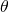# Gauss-Beam

The Gauss beam describes the shape of the incoming light at pixel level

# Gauss-points

4 points on the main optical axis of lenses:

Knowledge about the position of these 4 points is enough to calculate the position of an image point from the position of an object point.

That holds for single lens elements, but also for complex lens systems.

# Gaussian Optics

is another term for paraxial optics.

Carl Friedrich Gauss (1755-1855) laid in 1840 the foundations for this subject. In his work “Dioptrische Untersuchungen” Gauss showed, that the behaviour of any lens system can be determinedform the knowledge of it’s six cardinal points (also called Gauss-Points), namely two focal points, two nodal points (of unit angular magnification)  and two principal points (of unit linear magnification) .

Included in the paper were recipes for experimentally determining the positions of these points and iterative methods for calculating them in terms of the surface curvatures, separations and refractive indices of the lens system.

He explicitely mentioned  two equations where ray height r and ray angleof an input and output ray are linked, as used in the the ABCD-Matrix.But Matrix notation wasn’t known at that time. So instead he used an algorithm he learnt from Leonard Euler. It was a shorthand form of continuous fraction, now known as Gaussian Brackets.

It’s only allowed to use ABCD Matrices in the paraxial range, withThe anglesandare measured in radians!

For a table of ray transfer matrixes for simple optical components see ABCD matrix

# geometrical optics

Optical model for the description of the behaviour of lenses and other optical parts.
We can only use this model , if the wavelengths involved are very small compared to the equipment used for their study. Further the photon energy (see quantum theory) must be small compared with the energy sensitivity of the equipment.

Geometrical optics forgets about the wavelength and the photon character of light.
It ignores even what light is, just finds out, how it behaves.

Geometrical optics ignores diffraction and interference.

Geometrical optics can not be used for radio waves, because the wavelengths are larger than the equipment.

If wavelengths are in the region of the dimensions of the equipment (hard to reach with visible light, but easy with radio waves), and photo energies are negligibly small, then an approximation by study of the waves and disregarding quantum effects is appropriate called “classical theory of electromagnetic radiation”.

If wavelengths get very small, we can ignore the wave character of the light and photons have a large energy compared to the sensitivity of the equipment things get simple again, we get the simple photon picture.

The complete picture unifies everything into one model and is too complicated here.

compare to first order optics.

# gnomonic

gnomonic lenses (German : gnomonische Objektive) use an image mapping of the type[table caption=”gnomonic angles” width=”500″ colwidth=”50|50|50|50″ colalign=”center|center|center|center”]
weak,medium,strong,max
54,75,102,< 180
[/table]

Gnomonic lenses can provide a wide viewing angle , but are no fisheye lenses

see:
fisheye types